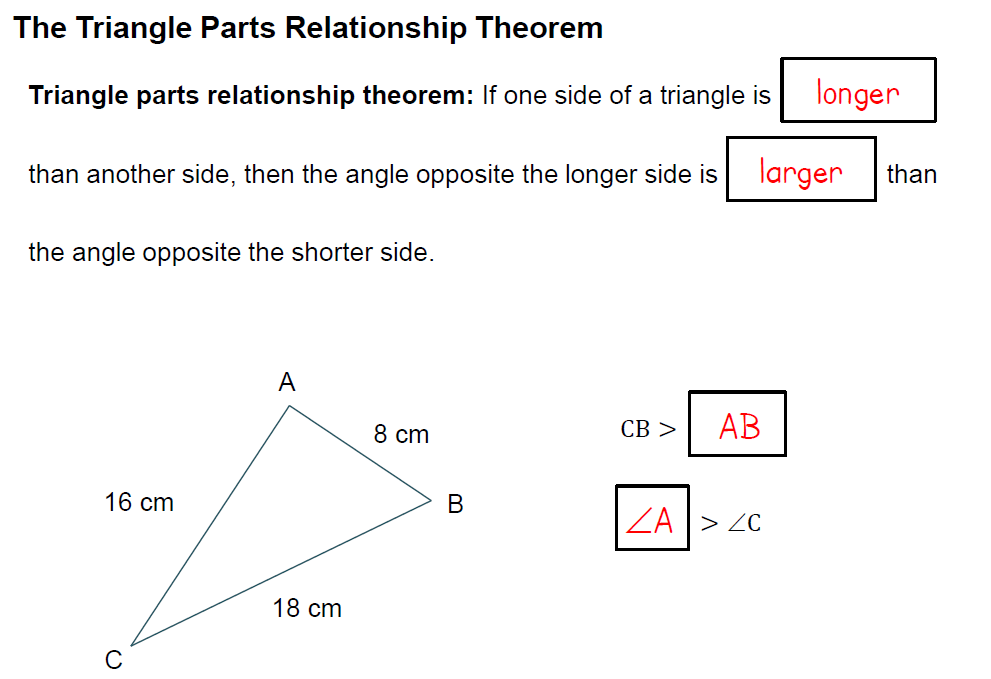# UNIT 4 LESSON 2: ISOSCELES TRIANGLES

## REMEMBER:Use the relationship between the side lengths and the angles opposite those sides. What are the smallest and largest angles of △LMN? The smallest angle of the triangle is ________.

Select all that apply
• A
• B
• C

The largest angle of the triangle is _______.

Select all that apply
• A
• B
• C

## PROPERTIES OF ISOSCELES TRIANGLES

WHICH OF THE FOLLOWING ARE TRUE ABOUT ISOSCELES TRIANGLES?

Select all that apply
• A
• B
• C
• D
• E
• F